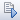»  Home »  Products »  Features »  Generalized SEM

## Generalized SEM

### Highlights

 Generalized linear responses Continuous—linear, gamma Binary—probit, logit, complementary log-log Count—Poisson, negative binomial, truncated Poisson Categorical—multinomial logit Ordered—ordered logit, ordered probit Censored continuous Fractional—beta Survival-time—exponential, loglogistic, Weibull, lognormal, gamma Multilevel data Nested: two levels, three levels, more levels Hierarchical Crossed Latent variables at different levels Random intercepts Random slopes (paths) Mixed models Multiple-group models Easily specify groups Constrain groups of parameters to be equal across groups Test for group invariance Meaning you can fit CFA with binary, count, and ordinal measurements Multilevel CFA Multilevel mediation Item response theory (IRT) Latent class analysis (LCA) Latent growth curves with repeated measurements of binary, count, and ordinal responses Selection models Endogenous treatment effects Multilevel survival models Survival models with latent predictors Any multilevel SEM with generalized linear responses Support for survey data Sampling weights Clustering Stratification Finite-population corrections

### Show me

Stata's sem command fits linear SEM.

Stata's gsem command fits generalized SEM, by which we mean (1) SEM with generalized linear response variables and (2) SEM with multilevel mixed effects, whether linear or generalized linear.

Generalized linear response variables mean you can fit logistic, probit, Poisson, multinomial logistic, ordered logit, ordered probit, beta, and other models. It also means measurements can be continuous, binary, count, categorical, ordered, fractional, and survival times.

Multilevel mixed effects means you can place latent variables at different levels of the data. You can fit models with fixed or random intercepts and fixed or random slopes.

Say we have a test designed to assess mathematical performance. The data record a set of binary variables measuring whether individual answers were correct. The test was administered to students at various schools.

We postulate that performance on the questions is determined by unobserved (latent) mathematical aptitude and by school quality, representing unmeasured characteristics of the school:

In the diagram, the values of the latent variable SchQual are constant within school and vary across schools.

We can fit the model from the path diagram by pressing. Results will appear on the diagram.

Or, we can skip the diagram and type the equivalent command

. gsem (       MathApt  -> q1 q2 q3 q4)
(SchQual[school] -> q1 q2 q3 q4), logit


Either way, we get the same results:

Generalized structural equation model                      Number of obs = 500

Log likelihood = -1348.3644

( 1)  [q1]SchQual[school] = 1
( 2)  [q2]MathApt = 1

Coefficient  Std. err.      z    P>|z|     [95% conf. interval]

q1
SchQual[school]            1  (constrained)

MathApt     5.277956   4.995708     1.06   0.291    -4.513451    15.06936
_cons     .0413352   .1770215     0.23   0.815    -.3056206    .3882909

q2
SchQual[school]      .600067   .3447607     1.74   0.082    -.0756516    1.275786

MathApt            1  (constrained)
_cons     -.449189   .1165887    -3.85   0.000    -.6776987   -.2206793

q3
SchQual[school]     .3999959   .3008142     1.33   0.184    -.1895891     .989581

MathApt     1.788696    1.10452     1.62   0.105    -.3761236    3.953516
_cons     .1485335   .1070996     1.39   0.165    -.0613779    .3584449

q4
SchQual[school]     .5925695     .34909     1.70   0.090    -.0916343    1.276773

MathApt     1.071626   .7310121     1.47   0.143    -.3611311    2.504384
_cons    -.3203425   .1152657    -2.78   0.005    -.5462592   -.0944258

var(Sch~l[sch~l])    .2483231     .24206                      .0367523    1.677838
var(MathApt)    .1050076   .1133871                      .0126501    .8716606


Math aptitude has a larger variance and loadings than school quality. Thus math aptitude is more important than school, although school is still important.

### Show me more

See Stata Structural Equation Modeling Reference Manual and especially see the introduction.

Don't miss the 28 worked examples demonstrating generalized SEM.